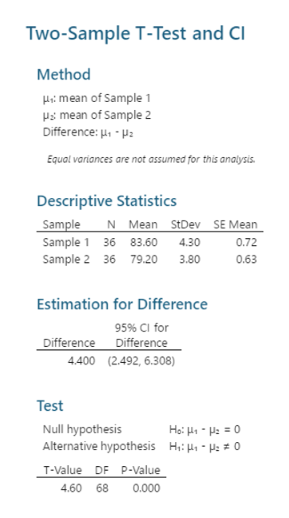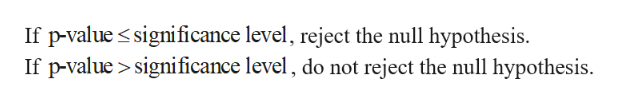# Two groups of students are given a problem solving a test and the results are compared. Determine whether there is no difference between the means. Use the p value mathod. mathemetics majors mean = 83.6 s1= 4.3 n1= 36 computer science majors mean = 79.2 s2= 3.8 n2= 36

Question
16 views

Two groups of students are given a problem solving a test and the results are compared. Determine whether there is no difference between the means. Use the p value mathod.

mathemetics majors mean = 83.6 s1= 4.3 n1= 36

computer science majors mean = 79.2 s2= 3.8 n2= 36

check_circle

Step 1

The hypotheses are given below:

Step 2

Step-by-step procedure to obtain the test statistic using MINITAB software:

• Choose Stat > Basic Statistics > 2 sample t.
• Choose Summarized data.
• In first, enter Sample size as 36, Mean as 6, Standard deviation as 4.3.
• In second, enter Sample size as 36, Mean as 2, Standard deviation as 3.8.
• Choose Options.
• In Confidence level, enter 95.
• In Alternative, select Not equal.
• Click OK in all the dialogue boxes.

Output obtained using MINITAB software is given below:help_outlineImage TranscriptioncloseTwo-Sample T-Test and Cl Method Hmean of Sample s mean of Sample 2 Difference: -pa Equal variances are not assumed for this analysis Descriptive Statistics Sample Sample 1 36 83.60 Sample 2 36 79.20 N Mean StDev SE Mean 4.30 0.72 3.80 0.63 Estimation for Difference 95% CI for Difference Difference 4.400 (2.492, 6.308) Test Ho: H-0 Null hypothesis Alternative hypothesis Hi 0 T-Value DF P-Value 4.60 68 0.000 fullscreen
Step 3

From the output, the test statistic is 4.60 and the p-value is 0.000.

Re...help_outlineImage TranscriptioncloseIf p-value significance level, reject the null hypothesis. If p-value significance level, do not reject the null hypothesis. fullscreen

### Want to see the full answer?

See Solution

#### Want to see this answer and more?

Solutions are written by subject experts who are available 24/7. Questions are typically answered within 1 hour.*

See Solution
*Response times may vary by subject and question.
Tagged in

### Hypothesis Testing